Next:Entropy of DegreeUp:Characterizations of Generalized Entropies

Entropy of Degree s

The following characterization of the measure (3.3) is due to Havrda and Charvát (1967) .

A function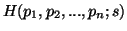will be said structural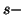entropy if

(i)is continuous in the region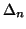for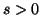.
(ii)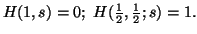(iii)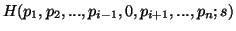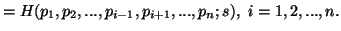(iv)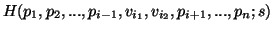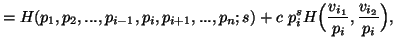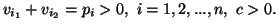Axioms (i)-(iv) determine the structuralentropy given by (3.3)

In the Rényi's case, Daróczy (1963)  restructured the axioms, while in the Havrda and Charvt's case, Daróczy (1970)  presented an alternative way to characterize the entropy of degree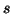. This is as follows:

Let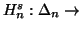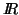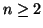be a real valued function satisfying: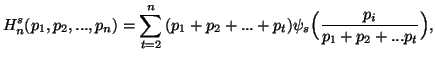where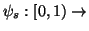is a function such that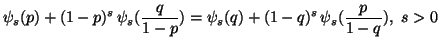for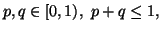with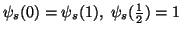, then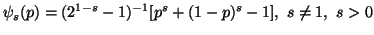(3.16)

and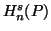is as given by (3.3).

The function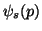given by (3.16) is famous as "information function of degree".

An alternative way to characterize the entropy of degreeis following the Chaundy and McLeod's (1961)  approach. This can be seen in Sharma and Taneja (1975) , and is as follows:

Letbe a real valued function satisfying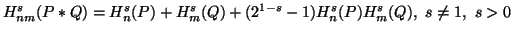for all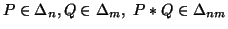, and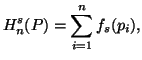where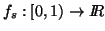is real valued continuous function with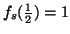. Thenis given by (3.3).

Some alternative approaches to characterize the measure (3.51) can be seen in Aczél and Daróczy (1975) , Mathai and Rathie (1975)  and Taneja (1979) .

It is worth emphasizing here that the measure (3.3), or better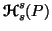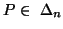satisfies some extra properties given below.

Property 3.18. We can write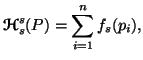where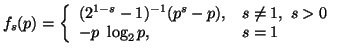Property 3.19. We can write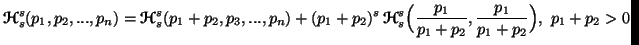Property 3.20. Let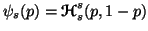, then

(i)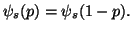(ii)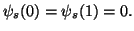(iii)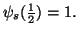(iv)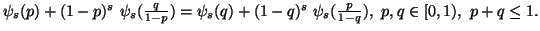(v)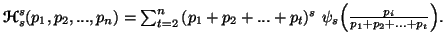Property 3.21. Let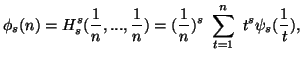with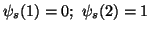. Then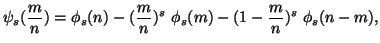for all rationals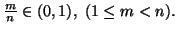Property 3.22. For all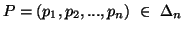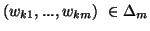,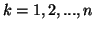, we have

(i)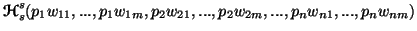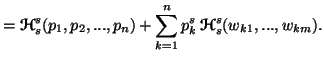(ii)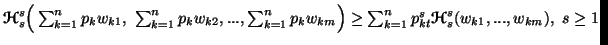Property 3.23. For all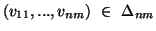, we have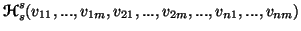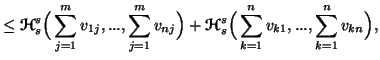for all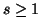.

21-06-2001
Inder Jeet Taneja
Departamento de Matemática - UFSC
88.040-900 Florianópolis, SC - Brazil